# 爬虫攻防之前端策略简析

## 1. 自定义字体形式

### 1.1 猫眼电影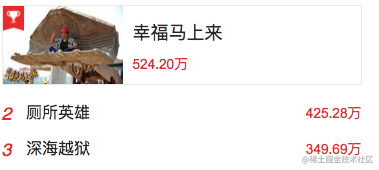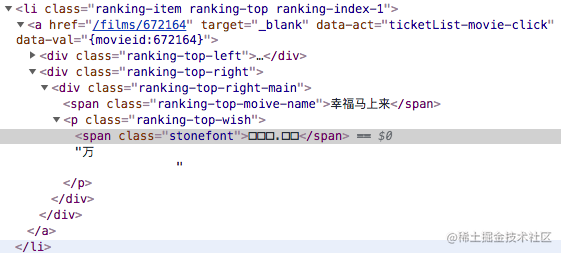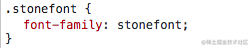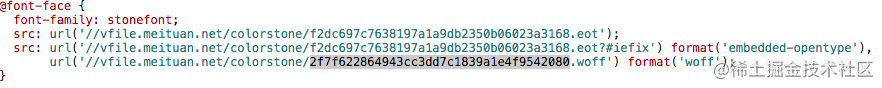woff字体是网页开放字体格式，详细可参见 MDN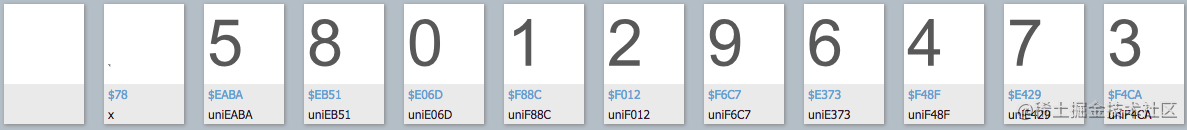``from fontTools.ttLib import TTFontfont = TTFont('/Users/coolcao/Downloads/b0a53bf9d791622d4681b8344fd118f92088.woff')font.saveXML('/Users/coolcao/maoyan2.xml')复制代码``

``<GlyphOrder>  <!-- The 'id' attribute is only for humans; it is ignored when parsed. -->  <GlyphID id="0" name="glyph00000"/>  <GlyphID id="1" name="x"/>  <GlyphID id="2" name="uniEABA"/>  <GlyphID id="3" name="uniEB51"/>  <GlyphID id="4" name="uniE06D"/>  <GlyphID id="5" name="uniF88C"/>  <GlyphID id="6" name="uniF012"/>  <GlyphID id="7" name="uniF6C7"/>  <GlyphID id="8" name="uniE373"/>  <GlyphID id="9" name="uniF48F"/>  <GlyphID id="10" name="uniE429"/>  <GlyphID id="11" name="uniF4CA"/></GlyphOrder>复制代码``

``<TTGlyph name="uniF4CA" xMin="0" yMin="-13" xMax="511" yMax="719">  <contour>    <pt x="130" y="201" on="1"/>    <pt x="145" y="126" on="0"/>    <pt x="216" y="60" on="0"/>    <pt x="270" y="60" on="1"/>    <pt x="332" y="60" on="0"/>    <pt x="417" y="146" on="0"/>    <pt x="417" y="270" on="0"/>    <pt x="378" y="309" on="1"/>    <pt x="337" y="349" on="0"/>    <pt x="277" y="349" on="1"/>    <pt x="251" y="349" on="0"/>    <pt x="215" y="339" on="1"/>    <pt x="225" y="416" on="1"/>    <pt x="239" y="415" on="1"/>    <pt x="296" y="415" on="0"/>    <pt x="385" y="474" on="0"/>    <pt x="385" y="535" on="1"/>    <pt x="385" y="583" on="0"/>    <pt x="322" y="646" on="0"/>    <pt x="268" y="646" on="1"/>    <pt x="217" y="646" on="0"/>    <pt x="149" y="584" on="0"/>    <pt x="139" y="518" on="1"/>    <pt x="51" y="533" on="1"/>    <pt x="67" y="623" on="0"/>    <pt x="124" y="670" on="1"/>    <pt x="182" y="719" on="0"/>    <pt x="266" y="719" on="1"/>    <pt x="324" y="719" on="0"/>    <pt x="374" y="693" on="1"/>    <pt x="423" y="669" on="0"/>    <pt x="476" y="581" on="0"/>    <pt x="476" y="485" on="0"/>    <pt x="426" y="410" on="0"/>    <pt x="377" y="388" on="1"/>    <pt x="440" y="373" on="0"/>    <pt x="511" y="281" on="0"/>    <pt x="511" y="211" on="1"/>    <pt x="511" y="118" on="0"/>    <pt x="374" y="-13" on="0"/>    <pt x="270" y="-13" on="1"/>    <pt x="175" y="-13" on="0"/>    <pt x="51" y="99" on="0"/>    <pt x="42" y="189" on="1"/>  </contour>  <instructions/></TTGlyph>复制代码``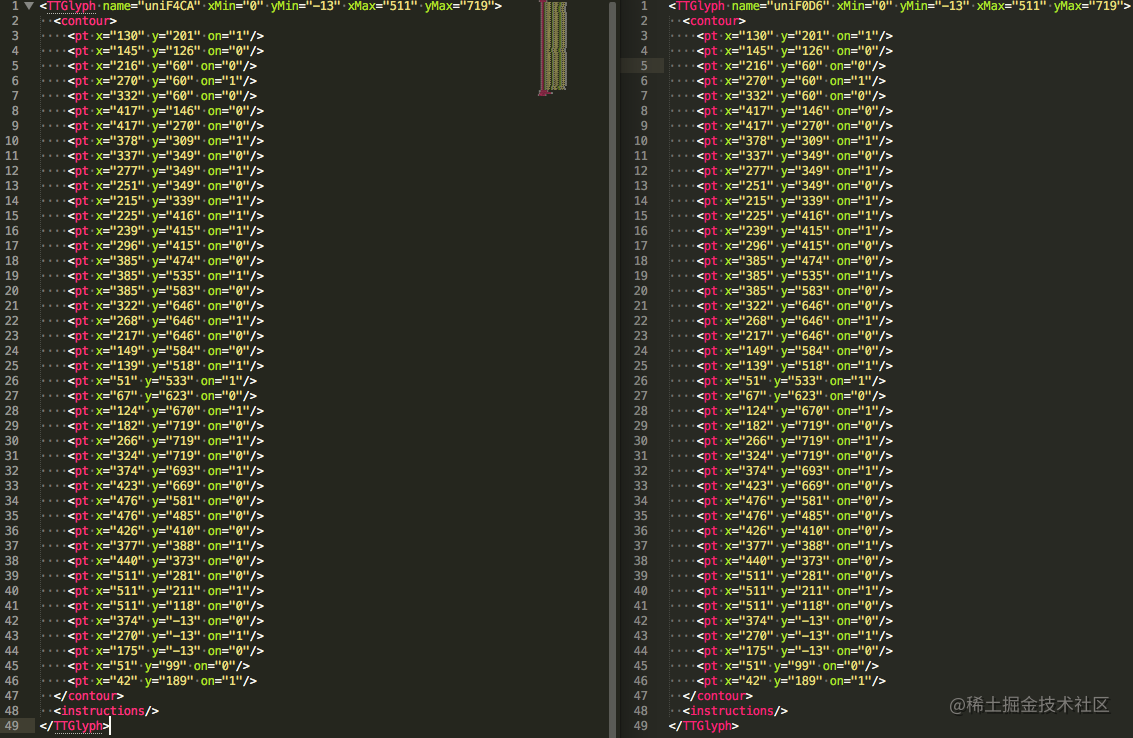### 1.2 去哪儿手机端网页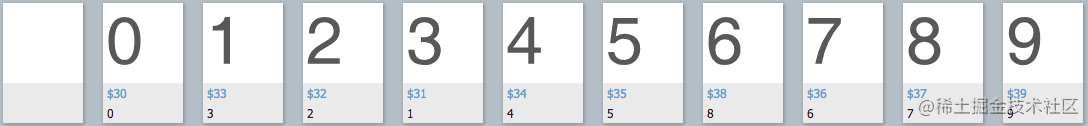### 1.3 起点中文网

``\$ node test.js94.37d821d821d821d821d821复制代码``

``<p>    <em>        <style>            @font-face {                 font-family: zxJBLkdl;                 src: url('https://qidian.gtimg.com/qd_anti_spider/zxJBLkdl.eot?') format('eot');                 src: url('https://qidian.gtimg.com/qd_anti_spider/zxJBLkdl.woff') format('woff'), url('https://qidian.gtimg.com/qd_anti_spider/zxJBLkdl.ttf') format('truetype');             }             .zxJBLkdl {                 font-family: 'zxJBLkdl' !important;                     display: initial !important;                 color: inherit !important;                 vertical-align: initial !important;             }        </style>        <span class="zxJBLkdl">&#100181;&#100184;&#100186;&#100181;&#100185;</span>    </em>    <cite>万字</cite><i>|</i><em><style>@font-face { font-family: zxJBLkdl; src: url('https://qidian.gtimg.com/qd_anti_spider/zxJBLkdl.eot?') format('eot'); src: url('https://qidian.gtimg.com/qd_anti_spider/zxJBLkdl.woff') format('woff'), url('https://qidian.gtimg.com/qd_anti_spider/zxJBLkdl.ttf') format('truetype'); } .zxJBLkdl { font-family: 'zxJBLkdl' !important;     display: initial !important; color: inherit !important; vertical-align: initial !important; }</style><span class="zxJBLkdl">&#100183;&#100185;&#100186;&#100181;&#100188;</span></em>    <cite>万总点击<span>&#183;</span>会员周点击        <style>        @font-face {            font-family: zxJBLkdl;            src: url('https://qidian.gtimg.com/qd_anti_spider/zxJBLkdl.eot?') format('eot');            src: url('https://qidian.gtimg.com/qd_anti_spider/zxJBLkdl.woff') format('woff'), url('https://qidian.gtimg.com/qd_anti_spider/zxJBLkdl.ttf') format('truetype');        }        .zxJBLkdl {            font-family: 'zxJBLkdl' !important;            display: initial !important;            color: inherit !important;            vertical-align: initial !important;        }        </style><span class="zxJBLkdl">&#100181;&#100185;&#100187;&#100184;</span></cite><i>|</i><em><style>@font-face { font-family: zxJBLkdl; src: url('https://qidian.gtimg.com/qd_anti_spider/zxJBLkdl.eot?') format('eot'); src: url('https://qidian.gtimg.com/qd_anti_spider/zxJBLkdl.woff') format('woff'), url('https://qidian.gtimg.com/qd_anti_spider/zxJBLkdl.ttf') format('truetype'); } .zxJBLkdl { font-family: 'zxJBLkdl' !important;     display: initial !important; color: inherit !important; vertical-align: initial !important; }</style><span class="zxJBLkdl">&#100179;&#100186;&#100181;&#100188;</span></em>    <cite>万总推荐<span>&#183;</span>周        <style>        @font-face {            font-family: zxJBLkdl;            src: url('https://qidian.gtimg.com/qd_anti_spider/zxJBLkdl.eot?') format('eot');            src: url('https://qidian.gtimg.com/qd_anti_spider/zxJBLkdl.woff') format('woff'), url('https://qidian.gtimg.com/qd_anti_spider/zxJBLkdl.ttf') format('truetype');        }        .zxJBLkdl {            font-family: 'zxJBLkdl' !important;            display: initial !important;            color: inherit !important;            vertical-align: initial !important;        }        </style><span class="zxJBLkdl">&#100188;&#100187;</span></cite></p>复制代码``

``<GlyphOrder>  <!-- The 'id' attribute is only for humans; it is ignored when parsed. -->  <GlyphID id="0" name=".notdef"/>  <GlyphID id="1" name="period"/>  <GlyphID id="2" name="zero"/>  <GlyphID id="3" name="one"/>  <GlyphID id="4" name="two"/>  <GlyphID id="5" name="three"/>  <GlyphID id="6" name="four"/>  <GlyphID id="7" name="five"/>  <GlyphID id="8" name="six"/>  <GlyphID id="9" name="seven"/>  <GlyphID id="10" name="eight"/>  <GlyphID id="11" name="nine"/></GlyphOrder>复制代码``

``<cmap_format_12 platformID="3" platEncID="10" format="12" reserved="0" length="148" language="0" nGroups="11">  <map code="0x18751" name="eight"/><!-- ???? -->  <map code="0x18753" name="two"/><!-- ???? -->  <map code="0x18754" name="five"/><!-- ???? -->  <map code="0x18755" name="three"/><!-- ???? -->  <map code="0x18756" name="zero"/><!-- ???? -->  <map code="0x18757" name="nine"/><!-- ???? -->  <map code="0x18758" name="six"/><!-- ???? -->  <map code="0x18759" name="four"/><!-- ???? -->  <map code="0x1875a" name="period"/><!-- ???? -->  <map code="0x1875b" name="one"/><!-- ???? -->  <map code="0x1875c" name="seven"/><!-- ???? --></cmap_format_12>复制代码``

## 5. 添加干扰字符并隐藏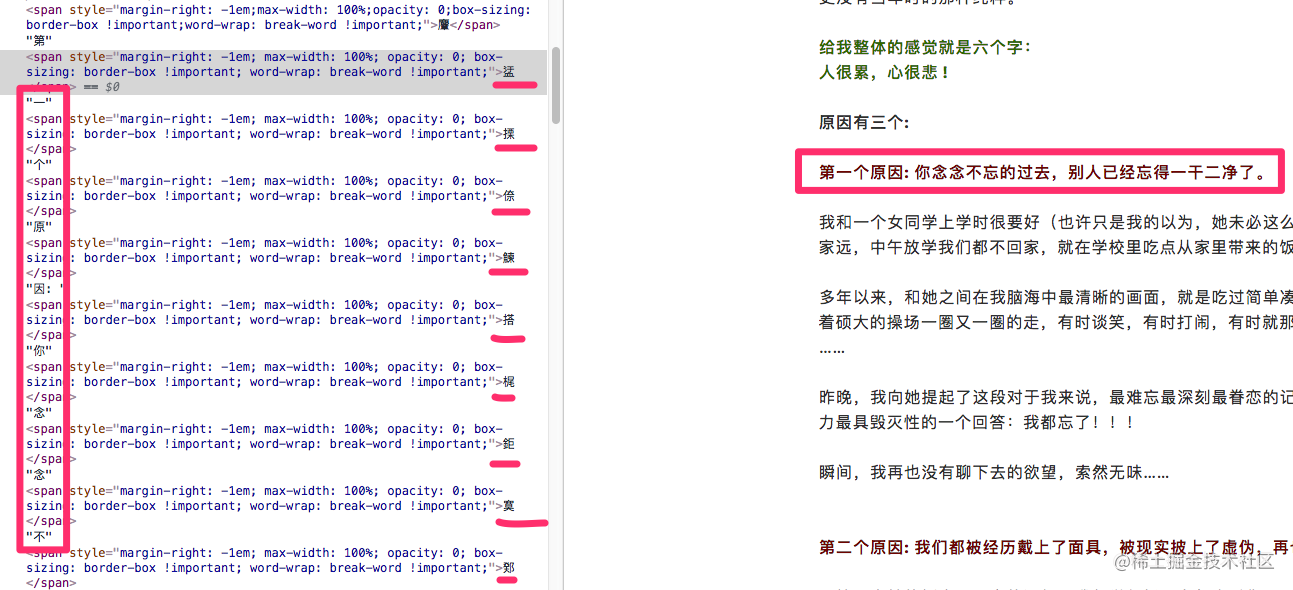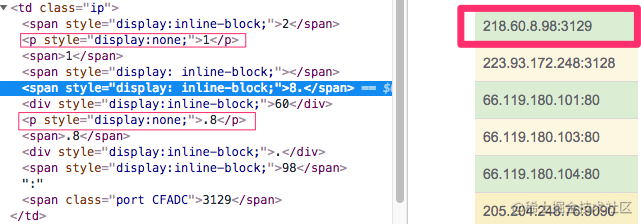## 6. 总结